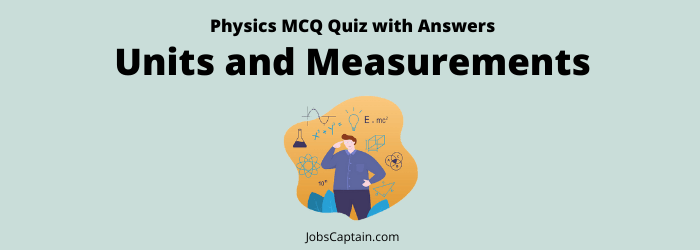# Physics: Units and Measurements MCQ Quiz 2Quiz Set 1 2

Question 1.  Which one of the following is not correctly matched?

(A) Celsius – Unit of heat

(B) Nautical miles – Unit of naval distance

(C) Horsepower – Unit of power

(D) Decibel – Unit of sound intensity

(A) Celsius – Unit of heat

Question 2.  Which one of the following is not the unit of heat?

(A) Watt

(B) Kilojoule

(C) Kilocalorie

(D) Calorie

(A) Watt

Question 3.  A distance of 1 km means _______.

(A) 100 cm

(B) 1000 m

(C) 1000 cm

(D) 100 m

(B) 1000 m

Question 4. One pikogram is equal to _______.

(A) 10–15 gram

(B) 10–12 gram

(C) 10–9 gram

(D) 10–6 gram

(B) 10–12 gram

Question 5. Pascal is a unit of measuring _________.

(A) Temperature

(B) Rainfall

(C) Pressure

(D) Humidity

(C) Pressure

Question 6.  Match List-I with List-II and select the correct answer using the code given below the lists.

 List-I List-II (a) Cusec 1. Pressure (b) Byte 2. Intensity of Earthquake (c) Richter 3. Rate of flow (d) Bar 4. Computer

Code

 (a) (b) (c) (d) (A) 3 4 1 2 (B) 4 3 2 1 (C) 3 4 2 1 (D) 1 2 3 4
(C) 3 4 2 1

Question 7.  Match List-I with List-II and select the correct answer using the code given below the lists.

 List-I (Quantity) List-II (Units) (a) High speed 1. Mach (b) Wavelength 2. Angstrom (c) Pressure 3. Pascal (d) Energy 4. Joule

Code

 (a) (b) (c) (d) (A) 2 1 4 3 (B) 1 2 3 4 (C) 1 2 4 3 (D) 2 1 3 4
(B) 1 2 3 4

Question 8.  Match the following.

 List-I List-II (a) Joule 1. Current (b) Ampere 2. Power (c) Watt 3. Work (d) Volt 4. Electric potential (e) Calorie 5. Heat

Code

 (a) (b) (c) (d) (e) (A) 1 3 2 4 5 (B) 4 3 2 1 5 (C) 1 2 3 4 5 (D) 3 1 2 4 5
(D) 3 1 2 4 5

Question 9.  Match List-I with List-II and select the correct answer using the code given below the lists.

 List-I (Units) List-II (Parametric quantities) (a) Watt 1. Heat (b) Knot 2. Navigation (c) Nautical mile 3. Speed of a ship (d) Calorie 4. Power

Code

 (a) (b) (c) (d) (A) 2 4 1 3 (B) 4 3 2 1 (C) 1 2 3 4 (D) 3 1 4 2
(B) 4 3 2 1

Question 10. Match List-I with List-II and select the correct answer using the code given below the lists.

 List-I (Physical quantities) List-II (Units) (a) Acceleration 1. Joule (b) Force 2. Newton second (c) Work done 3. Newton (d) Impulse 4. Metre/second

Code

 (a) (b) (c) (d) (A) 4 3 1 2 (B) 2 3 4 1 (C) 3 4 1 2 (D) 1 2 3 4
(A) 4 3 1 2

Question 11.  ‘Dobson’ unit is used for the measurement of ________.

(A) Measurement of Noise

(B) Thickness of Ozone layer

(C) Thickness of Diamond

(D) Thickness of Earth

(B) Thickness of Ozone layer

Question 12.  What is measured in Cusec?

(A) Quantity of water

(B) Flow of water

(C) Depth of water

(D) Purity of water

(B) Flow of water

Question 13.  One Nanometer is equal to ________.

(A) 10–3 m

(B) 10–10 m

(C) 10–6 m

(D) 10–9 m

(D) 10–9 m

Question 14.  The smallest unit of length is _______.

(A) Fermimetre

(B) Angstrom

(C) Nanometre

(D) Micron

(A) Fermimetre

Question 15. 1 barrel of oil is equals to which of the following?

(A) 201 litre

(B) 179 litre

(C) 159 litre

(D) 131 litre

(C) 159 litre

Question 16.  1 Kg/cm2  pressure is equivalent to _______.

(A) 100.0 bar

(B) 10.0 bar

(C) 1.0 bar

(D) 0.1 bar

(C) 1.0 bar

Question 17.  What is the unit of atmospheric pressure?

(A) Ohm

(B) Joule

(C) Knot

(D) Bar